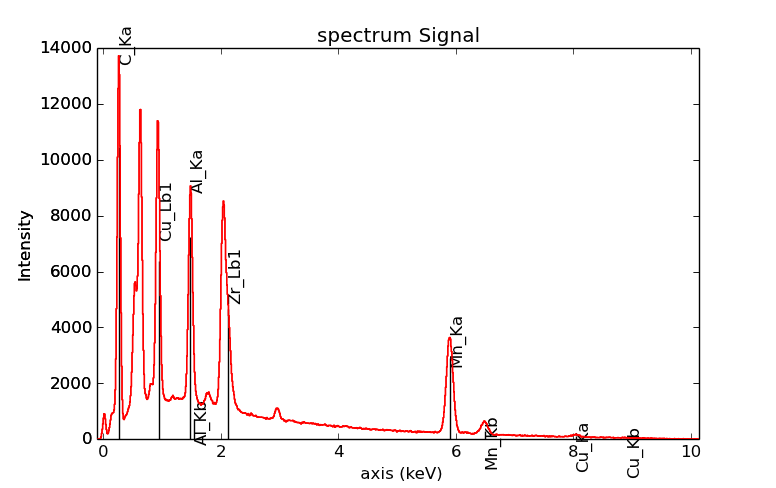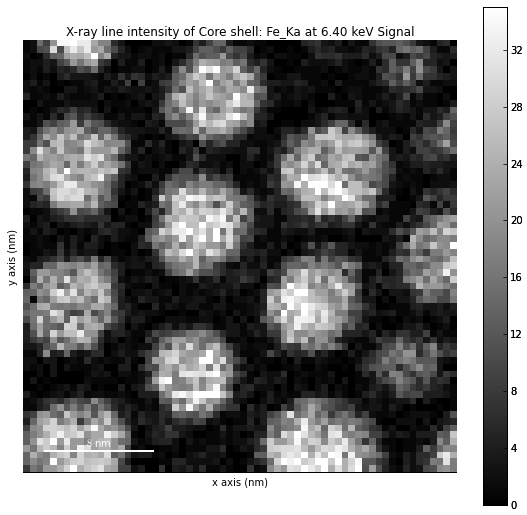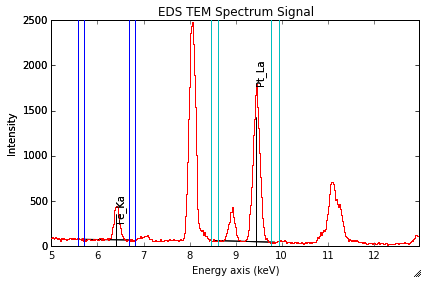# Energy-Dispersive X-Rays Spectrometry (EDS)¶

The methods described here are specific to the following signals:

This chapter described step by step the analysis of an EDS spectrum (SEM or TEM).

Note

```>>> from urllib import urlretrieve
>>> url = 'http://cook.msm.cam.ac.uk//~hyperspy//EDS_tutorial//'
>>> urlretrieve(url + 'Ni_superalloy_1pix.msa', 'Ni_superalloy_1pix.msa')
>>> urlretrieve(url + 'Ni_superalloy_010.rpl', 'Ni_superalloy_010.rpl')
>>> urlretrieve(url + 'Ni_superalloy_010.raw', 'Ni_superalloy_010.raw')
```

Note

The sample and the data used in this chapter are described in P. Burdet, et al., Acta Materialia, 61, p. 3090-3098 (2013) (see abstract).

All data are loaded with the `load()` function, as described in details in Loading files. HyperSpy is able to import different formats, among them ”.msa” and ”.rpl” (the raw format of Oxford Instrument and Brucker).

Here is three example for files exported by Oxford Instrument software (INCA). For a single spectrum:

```>>> s = hs.load("Ni_superalloy_1pix.msa")
>>> s
<Signal1D, title: Signal1D, dimensions: (|1024)>
```

For a spectrum image (The .rpl file is recorded as an image in this example, The method `as_signal1D()` set it back to a one dimensional signal with the energy axis in first position):

```>>> si = hs.load("Ni_superalloy_010.rpl").as_spectrum(0)
>>> si
<Signal1D, title: , dimensions: (256, 224|1024)>
```

For a stack of spectrum images (The “*” replace all chains of string, in this example 01, 02, 03,...):

```>>> si4D = hs.load("Ni_superalloy_0*.rpl", stack=True)
>>> si4D = si4D.as_signal1D(0)
>>> si4D
<Signal1D, title:, dimensions: (256, 224, 2|1024)>
```

### Microscope and detector parameters¶

First, the type of microscope (“EDS_TEM” or “EDS_SEM”) needs to be set with the `set_signal_type()` method. The class of the object is thus assigned, and specific EDS methods become available.

```>>> s = hs.load("Ni_superalloy_1pix.msa")
>>> s.set_signal_type("EDS_SEM")
>>> s
<EDSSEMSpectrum, title: Spectrum, dimensions: (|1024)>
```

or as an argument of the `load()` function:

```>>> s = hs.load("Ni_superalloy_1pix.msa", signal_type="EDS_SEM")
>>> s
<EDSSEMSpectrum, title: Spectrum, dimensions: (|1024)>
```

The main values for the microscope parameters are automatically imported from the file, if existing. The microscope and detector parameters are stored in stored in the `metadata` attribute (see Metadata structure). These parameters can be displayed as follow:

```>>> s = hs.load("Ni_superalloy_1pix.msa", signal_type="EDS_SEM")
├── Detector
│   └── EDS
│       ├── azimuth_angle = 63.0
│       ├── elevation_angle = 35.0
│       ├── energy_resolution_MnKa = 130.0
│       ├── live_time = 0.006855
│       └── real_time = 0.0
├── beam_current = 0.0
├── beam_energy = 15.0
└── tilt_stage = 38.0
```

These parameters can be set directly:

```>>> s = hs.load("Ni_superalloy_1pix.msa", signal_type="EDS_SEM")
```

or with the `set_microscope_parameters()` method:

```>>> s = hs.load("Ni_superalloy_1pix.msa", signal_type="EDS_SEM")
>>> s.set_microscope_parameters(beam_energy = 30)
```

or raising the gui:

```>>> s = hs.load("Ni_superalloy_1pix.msa", signal_type="EDS_SEM")
>>> s.set_microscope_parameters()
```

If the microscope and detector parameters are not written in the original file, some of them are set by default. The default values can be changed in the `Preferences` class (see preferences).

```>>> hs.preferences.EDS.eds_detector_elevation = 37
```

or raising the gui:

```>>> hs.preferences.gui()
```

### Energy axis¶

The main values for the energy axis are automatically imported from the file, if existing. The properties of the energy axis can be set manually with the `AxesManager`. (see Axis properties for more info):

```>>> si = hs.load("Ni_superalloy_010.rpl", signal_type="EDS_TEM").as_spectrum(0)
>>> si.axes_manager[-1].name = 'E'
>>> si.axes_manager['E'].units = 'keV'
>>> si.axes_manager['E'].scale = 0.01
>>> si.axes_manager['E'].offset = -0.1
```

or with the `gui()` method:

```>>> si.axes_manager.gui()
```

## Describing the sample¶

The description of the sample is stored in metadata.Sample (in the `metadata` attribute). It can be displayed as follow:

```>>> s = hs.datasets.example_signals.EDS_TEM_Spectrum()
├── description = FePt bimetallic nanoparticles
├── elements = ['Fe', 'Pt']
├── thickness = 100
└── xray_lines = ['Fe_Ka', 'Pt_La']
```

The following methods are either called “set” or “add”. When “set” methods erases all previously defined values, the “add” methods add the values to the previously defined values.

### Elements¶

The elements present in the sample can be defined with the `set_elements()` and `add_elements()` methods. Only element abbreviations are accepted:

```>>> s = hs.datasets.example_signals.EDS_TEM_Spectrum()
>>> s.set_elements(['Fe', 'Pt'])
└── elements = ['Cu', 'Fe', 'Pt']
```

### X-ray lines¶

Similarly, the X-ray lines can be defined with the `set_lines()` and `add_lines()` methods. The corresponding elements will be added automatically. Several lines per elements can be defined.

```>>> s = hs.datasets.example_signals.EDS_TEM_Spectrum()
>>> s.set_elements(['Fe', 'Pt'])
>>> s.set_lines(['Fe_Ka', 'Pt_La'])
├── elements = ['Fe', 'Pt']
└── xray_lines = ['Fe_Ka', 'Fe_La', 'Pt_La']
```

These methods can be used automatically, if the beam energy is set. The most excited X-ray line is selected per element (highest energy above an overvoltage of 2 (< beam energy / 2)).

```>>> s = hs.datasets.example_signals.EDS_SEM_Spectrum()
>>> s.set_elements(['Al', 'Cu', 'Mn'])
>>> s.set_microscope_parameters(beam_energy=30)
├── elements = ['Al', 'Cu', 'Mn']
└── xray_lines = ['Al_Ka', 'Cu_Ka', 'Mn_Ka']
```
```>>> s.set_microscope_parameters(beam_energy=10)
>>> s.set_lines([])
├── elements = ['Al', 'Cu', 'Mn']
└── xray_lines = ['Al_Ka', 'Cu_La', 'Mn_La']
```

A warning is raised, if setting a X-ray lines higher than the beam energy.

```>>> s = hs.datasets.example_signals.EDS_SEM_Spectrum()
>>> s.set_elements(['Mn'])
>>> s.set_microscope_parameters(beam_energy=5)
Warning: Mn Ka is above the data energy range.
```

### Element database¶

An elemental database is available with the energy of the X-ray lines.

```>>> hs.material.elements.Fe.General_properties
├── Z = 26
├── atomic_weight = 55.845
└── name = iron
>>> hs.material.elements.Fe.Physical_properties
└── density (g/cm^3) = 7.874
>>> hs.material.elements.Fe.Atomic_properties.Xray_lines
├── Ka
│   ├── energy (keV) = 6.404
│   └── weight = 1.0
├── Kb
│   ├── energy (keV) = 7.0568
│   └── weight = 0.1272
├── La
│   ├── energy (keV) = 0.705
│   └── weight = 1.0
├── Lb3
│   ├── energy (keV) = 0.792
│   └── weight = 0.02448
├── Ll
│   ├── energy (keV) = 0.615
│   └── weight = 0.3086
└── Ln
├── energy (keV) = 0.62799
└── weight = 0.12525
```

## Plotting¶

As decribed in visualisation, the `plot()` method can be used:

```>>> s = hs.datasets.example_signals.EDS_SEM_Spectrum()
>>> s.plot()
```

An example of plotting EDS data of higher dimension (3D SEM-EDS) is given in visualisation multi-dimension.

### Plot X-ray lines¶

New in version 0.8.

X-ray lines can be labbeled on a plot with `plot()`. The lines are either given, either retrieved from “metadata.Sample.Xray_lines”, or selected with the same method as `add_lines()` using the elements in “metadata.Sample.elements”.

```>>> s = hs.datasets.example_signals.EDS_SEM_Spectrum()
>>> s.plot(True)
```

Selecting certain type of lines:

```>>> s = hs.datasets.example_signals.EDS_SEM_Spectrum()
>>> s.plot(True, only_lines=['Ka','b'])
```EDS spectrum plot with a selection of line markers.

## Get lines intensity¶

```>>> from urllib import urlretrieve
>>> url = 'http://cook.msm.cam.ac.uk//~hyperspy//EDS_tutorial//'
>>> urlretrieve(url + 'core_shell.hdf5', 'core_shell.hdf5')
```

Note

The sample and the data used in this section are described in D. Roussow et al., Nano Lett, 10.1021/acs.nanolett.5b00449 (2015).

New in version 0.8.

The width of integration is defined by extending the energy resolution of Mn Ka to the peak energy (“energy_resolution_MnKa” in metadata).

```>>> s = hs.load('core_shell.hdf5')
>>> s.get_lines_intensity(['Fe_Ka'], plot_result=True)
```Iron map as computed and displayed by `get_lines_intensity`.

The X-ray lines defined in “metadata.Sample.Xray_lines” (see above) are used by default.

```>>> s = hs.load('core_shell.hdf5')
>>> s.set_lines(['Fe_Ka', 'Pt_La'])
>>> s.get_lines_intensity()
[<Signal2D, title: X-ray line intensity of Core shell: Fe_Ka at 6.40 keV, dimensions: (|64, 64)>,
<Signal2D, title: X-ray line intensity of Core shell: Pt_La at 9.44 keV, dimensions: (|64, 64)>]
```

The windows of integration can be visualised using `plot()` method

```>>> s = hs.datasets.example_signals.EDS_TEM_Spectrum()[5.:13.]
>>> s.plot(integration_windows='auto')
```

### Background subtraction¶

New in version 0.8.

The background can be subtracted from the X-ray intensities with the `get_lines_intensity()` method. The background value is obtained by averaging the intensity in two windows on each side of the X-ray line. The position of the windows can be estimated with the `estimate_background_windows()` method and can be plotted with the `plot()` method as follow. The integration windows are plotted with dashed lines.

```>>> s = hs.datasets.example_signals.EDS_TEM_Spectrum()[5.:13.]
>>> bw = s.estimate_background_windows(line_width=[5.0, 2.0])
>>> s.plot(background_windows=bw)
>>> s.get_lines_intensity(background_windows=bw, plot_result=True)
```EDS spectrum with background subtraction markers.

## Quantification¶

New in version 0.8.

One TEM quantification method (Cliff-Lorimer) is implemented so far.

Quantification can be applied from the intensities (background subtracted) with the `quantification()` method. The required k-factors can be usually found in the EDS manufacturer software.

```>>> s = hs.datasets.example_signals.EDS_TEM_Spectrum()
>>> kfactors = [1.450226, 5.075602] #For Fe Ka and Pt La
>>> bw = s.estimate_background_windows(line_width=[5.0, 2.0])
>>> intensities = s.get_lines_intensity(background_windows=bw)
>>> weight_percent = s.quantification(intensities, kfactors, plot_result=True)
Fe (Fe_Ka): Composition = 4.96 weight percent
Pt (Pt_La): Composition = 95.04 weight percent
```

The obtained composition is in weight percent. It can be changed transformed into atomic percent either with the option `quantification()`:

```>>> # With s, intensities and kfactors from before
>>> s.quantification(intensities, kfactors, plot_result=True,
>>>                  composition_units='atomic')
Fe (Fe_Ka): Composition = 15.41 atomic percent
Pt (Pt_La): Composition = 84.59 atomic percent
```

either with `weight_to_atomic()`. The reverse method is `atomic_to_weight()`.

```>>> # With weight_percent from before
>>> atomic_percent = hs.material.weight_to_atomic(weight_percent)
```